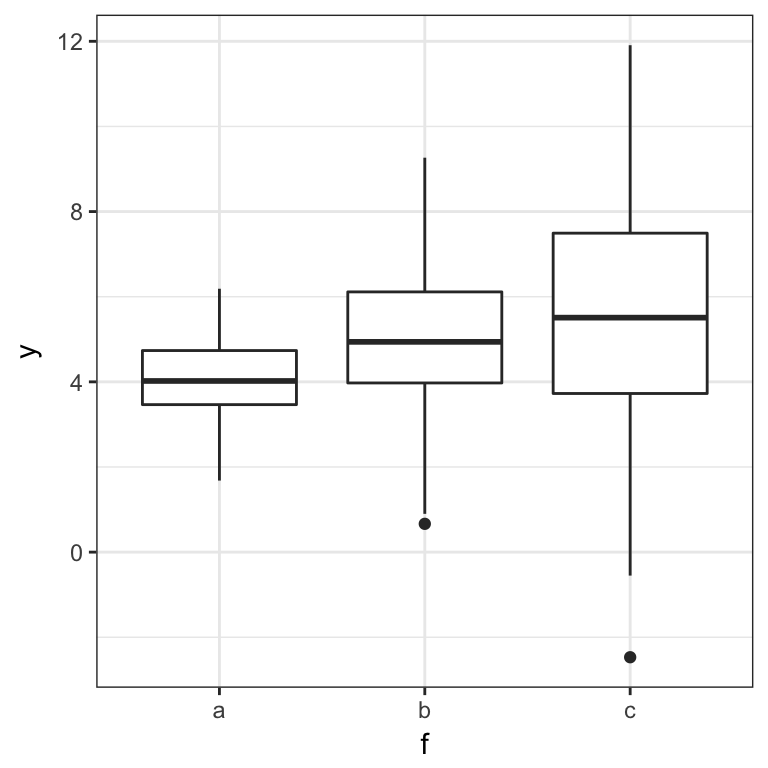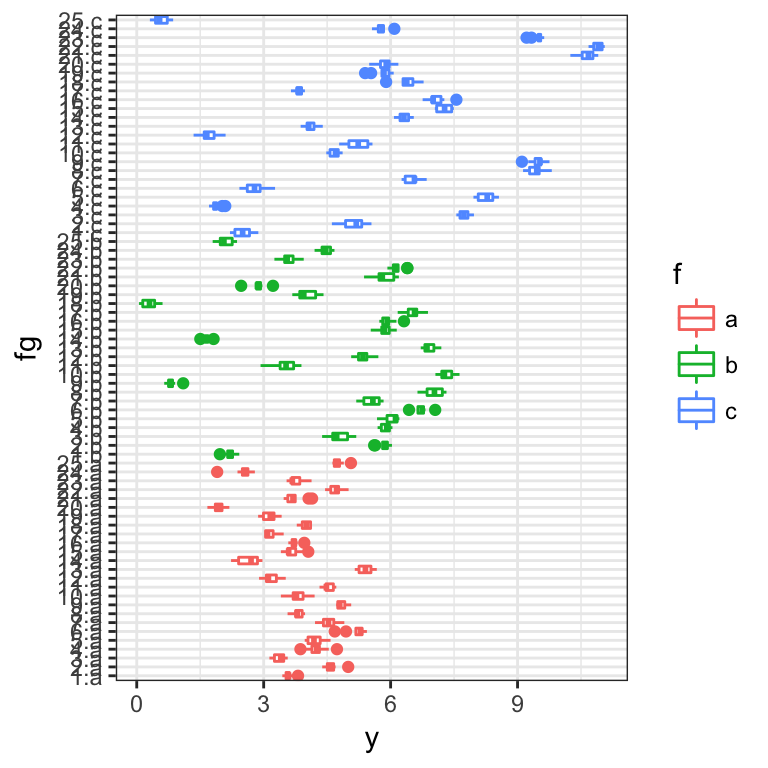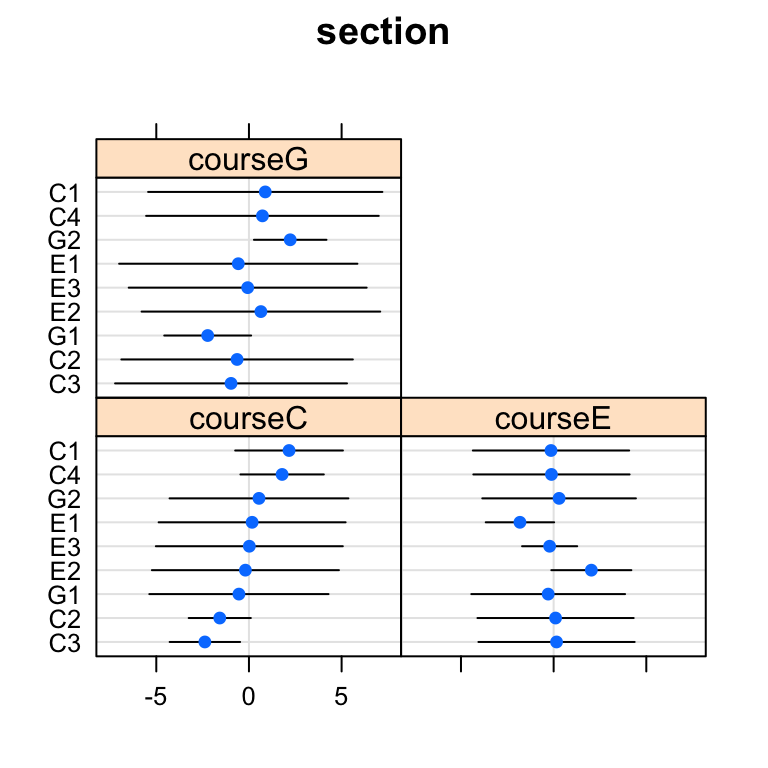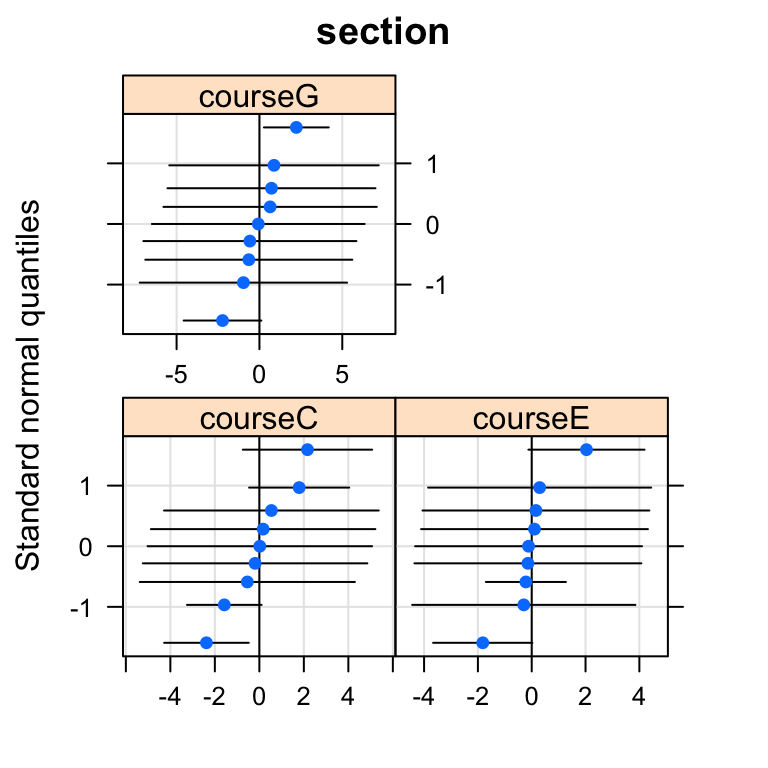Under construction!

library(lme4)
packageVersion("lme4")
##  '1.1.12'
library(nlme)
library(lattice)  ## for qqmath, dotplot
library("ggplot2"); theme_set(theme_bw())

A topic that has come up several times on the R list and elsewhere: (how) can one allow different variances among random effects within different categories? It turns out that this morphs into a couple of other topics of interest: narrowly, how can one fit a random effects model with diagonal variance-covariance model within a grouping factor? More generally, how can one fit random effects model with different variance-covariance structures in the random effects?

## Easy example: factor-specific residual variances

We don’t even need a mixed model for this one, we can just use generalized least squares (gls() in the nlme package). Suppose $\begin{split} Y_{ij} & = \delta_i + \epsilon_{ij} \\ \epsilon_{ij} & \sim \text{Normal}(0,\sigma^2_i) ; \end{split}$ that is, the residual variance differs by group.

set.seed(101)
dsd <- c(1,2,3)
d <- expand.grid(f=factor(letters[1:3]),rep=1:100)
delta <- 4:6
d$y <- rnorm(nrow(d),mean=delta[d$f],sd=dsd[d$f]) ggplot(d,aes(f,y))+geom_boxplot()library("nlme") m1 <- gls(y~f-1,data=d,weights=varIdent(form=~1|f)) We could look at the whole thing, but restricting ourselves for brevity to the fixed-effect parameter estimates and the estimated variances (parameterized as ratios of residual variances in groups $$2 \dots n$$ to the variance in the first group): coef(m1) ## fa fb fc ## 4.041845 5.015904 5.619241 summary(m1$modelStruct$varStruct) ## Variance function: ## Structure: Different standard deviations per stratum ## Formula: ~1 | f ## Parameter estimates: ## a b c ## 1.000000 1.905709 3.094312 The linear mixed-effect (lme()) and nonlinear mixed-effect (nlme()) functions in the nlme package also accept a weights/varIdent argument of this type, to specify R-side (residual) heteroscedasticity. ## Hard example: factor-specific random-effects variance ### Example 1 Now consider the two-level model $\begin{split} Y_{ijk} & = \delta_i + b_{ij} + \epsilon_{ijk} \\ b_{ij} & \sim \text{Normal}(0,\sigma^2_{b_j}) \\ \epsilon_{ijk} & \sim \text{Normal}(0,\sigma^2_0) ; \end{split}$ that is, now the structured variance is at the level of the among-group variance. This question is asked in http://thread.gmane.org/gmane.comp.lang.r.lme4.devel/7107, although it’s not 100% clear whether the question really refers to scenario #1 above. set.seed(101) dsd <- c(1,2,3) nblock <- 25 ntot <- 750 nfac <- 3 d <- expand.grid(f=letters[1:nfac],g=1:nblock,rep=seq(ntot/(nblock*nfac))) d$fg <- with(d,interaction(g,f))
delta <- 4:6
## have to be a bit careful to get everything in the right order here ...
u <- rnorm(nblock*nfac,mean=0,sd=rep(dsd,each=nblock))
d$y <- rnorm(nrow(d),mean=delta[d$f],sd=0.2)+u[d$fg] ggplot(d,aes(fg,y,colour=f))+geom_boxplot()+coord_flip()This can be done in lme: badly, as follows: lme(y~f-1,random=~f|g,data=d) ## Linear mixed-effects model fit by REML ## Data: d ## Log-restricted-likelihood: -67.13305 ## Fixed: y ~ f - 1 ## fa fb fc ## 3.881319 4.691717 5.973926 ## ## Random effects: ## Formula: ~f | g ## Structure: General positive-definite, Log-Cholesky parametrization ## StdDev Corr ## (Intercept) 0.8490982 (Intr) fb ## fb 2.0454336 -0.212 ## fc 2.9908972 -0.329 0.106 ## Residual 0.1898101 ## ## Number of Observations: 750 ## Number of Groups: 25 Or correctly: lme(y~f-1,random=list(g=pdDiag(~f)),data=d) ## Linear mixed-effects model fit by REML ## Data: d ## Log-restricted-likelihood: -69.06322 ## Fixed: y ~ f - 1 ## fa fb fc ## 3.881319 4.691717 5.973926 ## ## Random effects: ## Formula: ~f | g ## Structure: Diagonal ## (Intercept) fb fc Residual ## StdDev: 0.8483291 2.04441 2.989323 0.1898166 ## ## Number of Observations: 750 ## Number of Groups: 25 It can only be done in lme4 the bad way (at present). lmer(y~f-1+(f|g),data=d) ## Linear mixed model fit by REML ['lmerMod'] ## Formula: y ~ f - 1 + (f | g) ## Data: d ## REML criterion at convergence: 134.2661 ## Random effects: ## Groups Name Std.Dev. Corr ## g (Intercept) 0.8491 ## fb 2.0454 -0.21 ## fc 2.9909 -0.33 0.11 ## Residual 0.1898 ## Number of obs: 750, groups: g, 25 ## Fixed Effects: ## fa fb fc ## 3.881 4.692 5.974 We get approximately the right standard deviation values, but with bogus correlations (the off-diagonal elements must be zero in this case because of the way we defined the model …) W. Volterman points out that we can get the right variance components, although with some bogus information thrown in, by specifying an interaction of the nested blocks with the higher-level groups, as follows: lmer(y~f-1+(fg|g),data=d) However, for this example the model takes too long to run (see below for an example that does work, and a better way to do this). ### Example 2 A much smaller example proposed by WV: q3 <- structure(list(score = c(2L, 5L, 2L, 7L, 9L, 8L, 4L, 3L, 6L, 4L, 2L, 6L, 10L, 8L, 9L, 8L, 6L, 2L, 3L, 1L, 1L, 3L, 2L, 8L, 6L), course = structure(c(2L, 2L, 2L, 2L, 2L, 2L, 2L, 2L, 2L, 2L, 3L, 3L, 3L, 3L, 3L, 1L, 1L, 1L, 1L, 1L, 1L, 1L, 1L, 1L, 1L ), .Label = c("C", "E", "G"), class = "factor"), section = structure(c(5L, 5L, 5L, 6L, 6L, 7L, 7L, 7L, 7L, 7L, 8L, 8L, 9L, 9L, 9L, 1L, 2L, 2L, 2L, 2L, 3L, 3L, 3L, 4L, 4L), .Label = c("C1", "C2", "C3", "C4", "E1", "E2", "E3", "G1", "G2"), class = "factor")), .Names = c("score", "course", "section"), class = "data.frame", row.names = c(NA, -25L)) q3.lme4<-lmer(score~0+course+(0+course|section),data=q3, control=lmerControl(check.nobs.vs.nRE="ignore")) ## Warning in checkConv(attr(opt, "derivs"), opt$par, ctrl = control
## $checkConv, : unable to evaluate scaled gradient ## Warning in checkConv(attr(opt, "derivs"), opt$par, ctrl = control
## $checkConv, : Model failed to converge: degenerate Hessian with 1 negative ## eigenvalues summary(q3.lme4) ## Linear mixed model fit by REML ['lmerMod'] ## Formula: score ~ 0 + course + (0 + course | section) ## Data: q3 ## Control: lmerControl(check.nobs.vs.nRE = "ignore") ## ## REML criterion at convergence: 105.4 ## ## Scaled residuals: ## Min 1Q Median 3Q Max ## -1.2852 -0.6592 -0.1555 0.6728 1.6392 ## ## Random effects: ## Groups Name Variance Std.Dev. Corr ## section courseC 6.657 2.580 ## courseE 4.648 2.156 -0.08 ## courseG 11.133 3.337 0.31 0.20 ## Residual 3.282 1.812 ## Number of obs: 25, groups: section, 9 ## ## Fixed effects: ## Estimate Std. Error t value ## courseC 4.769 1.438 3.318 ## courseE 5.247 1.384 3.790 ## courseG 6.555 2.499 2.623 ## ## Correlation of Fixed Effects: ## coursC coursE ## courseE 0.000 ## courseG 0.000 0.000 ## convergence code: 0 ## unable to evaluate scaled gradient ## Model failed to converge: degenerate Hessian with 1 negative eigenvalues (x1<-ranef(q3.lme4)) ##$section
##        courseC    courseE     courseG
## C1  2.16396546 -0.1421228  0.87972396
## C2 -1.57514553  0.1034508 -0.64034907
## C3 -2.37843840  0.1562087 -0.96691435
## C4  1.78961847 -0.1175368  0.72753945
## E1  0.17108276 -1.8186815 -0.57620769
## E2 -0.19142031  2.0348781  0.64470466
## E3  0.02033755 -0.2161967 -0.06849697
## G1 -0.54124631 -0.2944998 -2.22653229
## G2  0.54124631  0.2944998  2.22653229

Plots include estimates which should be zero (but aren’t: they could be eliminated, with enough hacking around).

dotplot(ranef(q3.lme4,condVar=TRUE))
## $sectionqqmath(ranef(q3.lme4,condVar=TRUE)) ##$sectionWe can fit the same model with lme()

q3.nlme <- lme(score~0+course,random=~0+course|section,data=q3)
summary(q3.nlme)
## Linear mixed-effects model fit by REML
##  Data: q3
##       AIC      BIC    logLik
##   125.387 136.2974 -52.69349
##
## Random effects:
##  Formula: ~0 + course | section
##  Structure: General positive-definite, Log-Cholesky parametrization
##          StdDev   Corr
## courseC  2.580088 coursC coursE
## courseE  2.155847 0
## courseG  3.336581 0      0
## Residual 1.811521
##
## Fixed effects: score ~ 0 + course
##            Value Std.Error DF  t-value p-value
## courseC 4.769270  1.437556  6 3.317624  0.0161
## courseE 5.246728  1.384453  6 3.789748  0.0091
## courseG 6.554693  2.499413  6 2.622493  0.0395
##  Correlation:
##         coursC coursE
## courseE 0
## courseG 0      0
##
## Standardized Within-Group Residuals:
##        Min         Q1        Med         Q3        Max
## -1.2851952 -0.6591829 -0.1554550  0.6727893  1.6392136
##
## Number of Observations: 25
## Number of Groups: 9
x2<-ranef(q3.nlme)

Estimated random effects are roughly the same (at least, the ones with any meaning are)

colGrp <- substr(names(x2),7,7)
rowGrp <- substr(rownames(x2),1,1)
x2mat <- as.matrix(x2)
x2mat[rowGrp[row(x2)]!=colGrp[col(x2)]] <- NA
x2 <- as.data.frame(x2mat)
all(abs(na.omit(unlist(x1[]-x2)))<4e-6)
##  FALSE

We can do better with the development version of lme4 and some hacking, fitting by wrapping deviance function, setting relevant $$\theta$$ parameters to zero. In the long run it would be better (more efficient) to change Lind/Lambdat in mkReTrms.

If the lower triangle of the Cholesky factor of a $$2 \times 2$$ matrix is $$\{\theta_1,\theta_2,\theta_3\}$$ then the matrix is \[ \left( \begin{array}{cc} \theta_1^2 & \theta_1 \theta_2 \\ \theta_1 \theta_2 & \thet